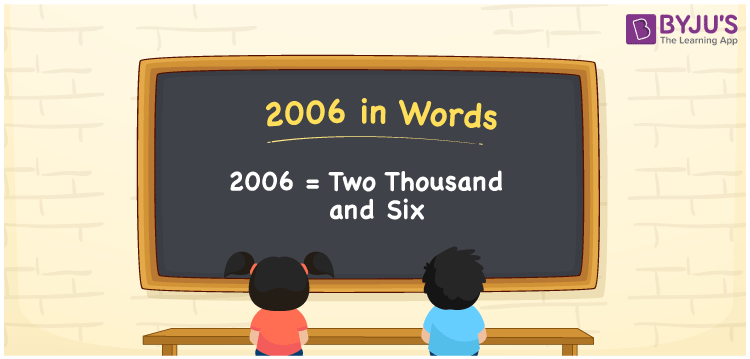# 2006 in Words

The number 2006 represents “Two Thousand and Six”. Since the number 2006 is used to count things and express values, we can say that 2006 is a cardinal number. Converting the number 2006 to words can be done using the place value system. The price of the shirt is Rs. 2006. This can be written as “The price of the shirt is Rs. Two Thousand and Six”. In this article, let us discuss how to write 2006 in words as well as the technique used in detail.

 2006 in Words: Two Thousand and Six. Two Thousand and Six in Numerical Form: 2006.

## 2006 in English Words## How to Write 2006 in Words?

The number 2006 in words is written using the place value of the number. Below is the place value table for the number 2006.

 Thousands Hundreds Tens Ones 2 0 0 6

The expanded form of 2006 is as follows:

= 2 × Thousand + 0 × Hundred + 0 × Ten + 6 × One

= 2 × 1000 + 6 × 1

= 2000 + 6

= 2006

= Two thousand and six

Hence, 2006 in words is two thousand and six.

2006 in words – Two thousand and six

Is 2006 an odd number? – No

Is 2006 an even number? – Yes

Is 2006 a perfect square number? – No

Is 2006 a perfect cube number? – No

Is 2006 a prime number? – No

Is 2006 a composite number? – Yes

## Frequently Asked Questions on 2006 in Words

Q1

### How to spell 2006?

2006 in words is two thousand and six.

Q2

### Simplify 2000 + 6, and express in words.

Simplifying 2000 + 6, we get 2006. Hence, 2006 in words is two thousand and six.

Q3

### Is 2006 an odd number?

No, 2006 is not an odd number.